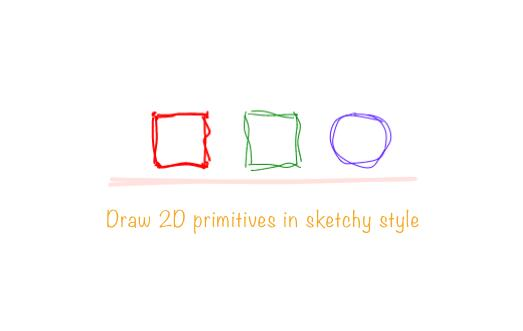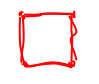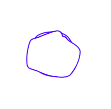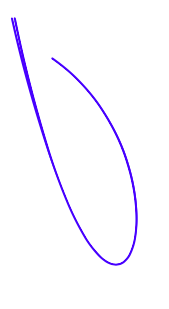/ Images

# Draw 2D primitives in sketchy style with React## react-handy-renderer

✏️   Draw 2D primitives in sketchy style with React

## Install

``````npm install react-handy-renderer
``````

## Usage

`react-handy-renderer` exposes a set of five primitives:

• Rectangle
• RoundedRect
• Line
• Curve
• Ellipse

and one wrapper component called Paper which serves as a canvas on which these primitives are drawn.

Here is a basic example -

``````import React from 'react';
import { render, Paper, Ellipse } from 'react-handy-renderer';

function Sketch() {
return (
<Paper bowing={0.2} width={600} height={500}>
<Ellipse
position={{ x: 480, y: 100 }}
width={50}
height={50}
color="mistyrose"
weight={0.9}
roughness={3}
/>
</Paper>
);
}

render(<Sketch />, document.getElementById('canvas-container'));
``````

## Examples

### RoundedRect``````<RoundedRect
position={{ x: 300, y: 100 }}
width={50}
height={50}
color="red"
weight={2}
roughness={1.8}
/>
``````

### Rectangle``````<Rectangle
position={{ x: 390, y: 100 }}
width={50}
height={50}
color="green"
weight={1}
roughness={2.3}
/>
``````

### Ellipse``````<Ellipse
position={{ x: 480, y: 100 }}
width={50}
height={50}
color="blue" // or color={[10, 10, 10, 20]}
weight={0.9}
roughness={3}
bowing={9}
maxOffset={10}
/>
``````

### Line``````<Line
from={{ x: 230, y: 140 }}
to={{ x: 530, y: 140 }}
weight={4}
color="mistyrose"
roughness={2}
/>
``````

### Curve``````<Curve
c1={{ x: 30, y: 50 }}
c2={{ x: 70, y: 90 }}
c3={{ x: 130, y: 510 }}
c4={{ x: 230, y: 200 }}
color="blue"
weight={2}
roughness={2}
/>
``````

## Usage with ReactDOM renderer

You can also render the primitives when working with ReactDOM as this just renders to a canvas node.

Example -

``````import React, { Component } from 'react'
import {
render as create,
Paper,
Rectangle,
Main,
Ellipse,
Line,
RoundedRect,
Curve,
} from 'react-handy-renderer'

function Sketch() {
return (
<Paper bowing={0.2} width={500} height={500}>
<Line
from={{x: 10, y: 40}}
to={{x: 40, y: 60}}
weight={10}
color="red"
/>
</Paper>
)
}

// Renders the canvas node
create(<Sketch />, document.getElementById('root'))

// create function has already rendered the canvas node.
// So now you can continue to use React with ReactDOM as you used to.
// Make changes directly to the <Sketch /> component.
class App extends Component {
render() {
return (
<div>
REACT HANDY RENDERER
</div>
)
}
}

export default App
``````

## API

### Paper

Wrapper component for creating the canvas.

Props

• `width` - width of the canvas

• `height` - height of the canvas

• `roughness` - Number value for changing the roughness of shapes

• `bowing` - Number value for changing the bowing of lines

• `maxOffset` - Number value for giving coordinates an offset,

Example

### Curve

Props

• `c1` - First control point's `x` and `y` coordinate values

• `c2` - Second control point's `x` and `y` coordinate values

• `c3` - Third control point's `x` and `y` coordinate values

• `c4` - Fourth control point's `x` and `y` coordinate values

• `roughness` - Number value for changing the roughness

• `bowing` - Number value for changing the bowing of lines

• `maxOffset` - Number value for giving coordinates an offset,

• `color` - String value for color

Example

### Ellipse

Props

• `position` - It is an object that takes `x` and `y` coordinate values.

• `width` - defines width

• `height` - defines height

• `color` - sets the color for stroke

• `weight` - sets the stroke thickness

• `roughness` - Number value for changing the roughness

• `bowing` - Number value for changing the bowing of lines

• `maxOffset` - Number value for giving coordinates an offset,

• `fill` - sets the fill color for filling the ellipse

Example

### Rectangle

Props

• `position` - It is an object that takes `x` and `y` coordinate values.

• `width` - defines width

• `height` - defines height

• `color` - sets the color for stroke

• `weight` - sets the stroke thickness

• `roughness` - Number value for changing the roughness

• `bowing` - Number value for changing the bowing of lines

• `maxOffset` - Number value for giving coordinates an offset,

Example

### RoundedRect

Props

• `position` - It is an object that takes `x` and `y` coordinate values.

• `width` - defines width

• `height` - defines height

• `radius` - sets the radius

• `color` - sets the color for stroke

• `weight` - sets the stroke thickness

• `roughness` - Number value for changing the roughness

• `bowing` - Number value for changing the bowing of lines

• `maxOffset` - Number value for giving coordinates an offset,

Example

### Line

props

• `from` - An object that takes `x1` and `y1` coordinate value.

• `to` - An object that takes `x2` and `y2` value

• `color` - sets the color for stroke

• `weight` - sets the stroke thickness

• `roughness` - Number value for changing the roughness

• `bowing` - Number value for changing the bowing of lines

• `maxOffset` - Number value for giving coordinates an offset,

Example

## Todo

• [ ] Define hachures
• [ ] More examples